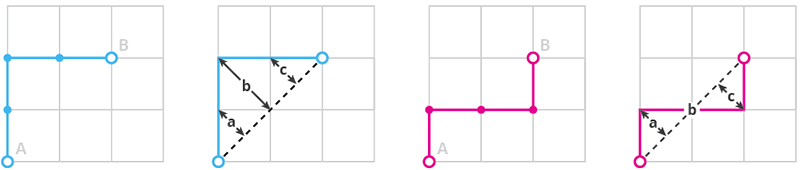SEARCH AND PRESS ENTER
Maya API / 14 Jun 2016
Maya API: Shortest path between vertices

Have you ever wondered how to find the shortest path between vertices on mesh? I have. Here’s the code.

I have recently decided to update few of my custom Maya tools from MEL to API. The most useful one, Planarize Edge Tool, was frankensteined from ZenTools, which contains all sort of useful functions for working with vertices. Now that I moved to C++, I had to come up with my own solution for finding the shortest path between multiple vertices. Before I could  do that, I needed to cover a simple two-point scenario.

### Shortest path between two vertices

Lets say we have even square mesh and vertices A and B. Since all edges have the same length (weight), the shortest path between vertices A and B will be the one with least iterations. In the following example it is four. In every iteration the path grafts to all connected points, increasing the search radius until the end vertex is reached.As you probably noticed, this method itself does’t produce a single path, rather a whole tree. To narrow it down we have to apply some rule. Following illustration shows two paths, both are results of the previous example. By measuring deviation of each path from a straight line between the two end-vertices, we can figure out which one is the most “direct” path. As you can see total deviation (a+b+c) is smaller with the second path. The deciding factor could be actually anything – corner angle, edge length, vertex index or color.### Implementation

We begin by initializing the storage variables and by extracting  the base mesh and the end vertices from active selection.

Now we have to convert the vertex data in correct format. For certain operations we’ll use vertices in form of a component object, in other cases it is easier to work with vertex indices in numeric format.

### ‘Inception’

The extendPath function does all the magic thanks to MItMeshVertex::getConnectedVertices() method, which lists point on the other side of each edge connected to given vertex. In the first loop we call this function using the start point, in every next round it uses vertices collected in the previous iteration. This repeats until we reach the target vertex. It is a good idea to limit the number of iterations to number of all mesh vertices, to prevent infinite loop. Notice how we use the path deviation to choose between multiple paths pointing to the same vertex.

This function generates path in a string format containing vertex indices separated by semilocon e.g. “1;2;3;4;5;6”. For a bit more API friendly result use the following function to converted it into a numeric array.

### Conclusion

This fairly simple code is a great entry point for more complex solutions. By comparing paths generated by this method you can sort multiple vertices along an edge and find  the shortest path between multiple vertices. Watch out for my future blog posts, it will definitely appear there.

1.#### Ravshan Gaziev

24 Apr 2022 - 1:17 pm

You can use just “polySelect” func. It will be much more short and faster

•#### Stepan Jirka

25 Apr 2022 - 8:17 am

Hi Ravshan,

thank you for your suggestion – you might be right. I was looking for a solution that would work directly with C++ API without the need to call MEL or Python command.